﻿ Identification of Crack in Beam Using Hilbert – Huang Transform

### Identification of Crack in Beam Using Hilbert – Huang Transform

Mangesh Dilip Ratolikar, M Chandra Sekhar ReddyOPEN ACCESSPEER-REVIEWED

## Identification of Crack in Beam Using Hilbert – Huang Transform

Mangesh Dilip Ratolikar1,, M Chandra Sekhar Reddy1

1Department of Mechanical Engineering, College of Engineering, Osmania University, Hyderabad, India

### Abstract

In this paper, investigations on vibrations of cracked beam structures and methodology for crack identification have been addressed. Here, the crack is modelled as transverse crack and it is considered as a small element and is later assembled with the other discretized elements using FEM techniques. Using this model, vibration analysis of simply supported, fixed-fixed, free-free and cantilever solid rectangular beams, with crack is carried out. The fundamental vibration modes of damaged beam are analyzed using Hilbert-Huang transform (HHT). The location of crack is determined by the sudden changes in the spatial variation of the transformed response. The results in both the simulation mode as well as experimental mode show that, HHT is an effective tool for the crack detection. The proposed technique is validated both analytically and experimentally thus the results shown have a good agreement with the established model.

### At a glance: Figures

• Ratolikar, Mangesh Dilip, and M Chandra Sekhar Reddy. "Identification of Crack in Beam Using Hilbert – Huang Transform." Journal of Mechanical Design and Vibration 2.4 (2014): 87-93.
• Ratolikar, M. D. , & Reddy, M. C. S. (2014). Identification of Crack in Beam Using Hilbert – Huang Transform. Journal of Mechanical Design and Vibration, 2(4), 87-93.
• Ratolikar, Mangesh Dilip, and M Chandra Sekhar Reddy. "Identification of Crack in Beam Using Hilbert – Huang Transform." Journal of Mechanical Design and Vibration 2, no. 4 (2014): 87-93.

 Import into BibTeX Import into EndNote Import into RefMan Import into RefWorks

### 1. Introduction

Any changes to the material and geometric properties of a system, comprisingof changes to the boundary conditions and system connectivity, which adversely affect the current and future performance of that system is considered as damage. The occurrence of damage in a structure produces changes in its global dynamic characteristics such as its natural frequencies, mode shapes, modal damping, modal participation factors, impulse response and frequency response functions thereby weakening the structural strength.

Structural damage identification using dynamic parameters of the structure has become an important research area. M. Bezhad et al.  in their paper presented a simple method for crack detection of multiple edge cracks in Euler – Bernoulli beams having two different types of cracks based on energy equations. Crack were modelled as a massless rotational springs using Linear Elastic Fracture Mechanics. X. B. Lu et al.  introduced a two-step approach based on mode shape curvature and response sensitivity analysis for crack identification in beam structure. The difference between the mode shape curvature of cracked beam before and after crack determines crack location. A. P. Adewuyi et al.  analyzed performance evaluation for practical civil structural health monitoring by using displacement modes from accelerometers and long gauge distributed strain measurements through computer simulation and experimental investigation. P. F. Rizo et al.  described about the measurement of flexural vibrations of a cantilever beam with rectangular cross section having a transverse surface crack extending uniformly along the width of the beam to relate the measured vibration modes to the crack location and depth. M. Cao et al.  studied fundamental mode shape and static deflection for damage identification in cantilever beams. The results proposed provides a theoretical basis for optimal use for damage identification in cantilever beams.

The dynamic responses of the system are used for crack prediction. D. Guo et al.  discussed startup transient response of a rotor with a propagating transverse crack using Hilbert-Huang transform. The rotor with a growing crack was modelled by finite element method. The rotating frequency vibration components were studied when the frequency was reached peak and decayed during startup process. The demonstration of HHT in unsteady transient case gave an idea of HHT and its limits. T. R. Babu et al.  analyzed Hilbert-Huang transform being applied to transient response of a cracked rotor. It was established that, HHT is comparatively better than continuous wavelet transform and fast Fourier transform. B. Li et al.  developed the novel crack identification method, HHT and its algorithm. The validity of mentioned method was confirmed with an experiment. The conclusion was quite helpful in carrying this research work. N. E. Huang, S. S. P. Shen  in their book explained the evolution of HHT and the algorithm for the transform. The development of HHT and procedure of applying the transform to any data was found by the author himself, N. E. Huang.

In the current research, a number of research papers published till now have been studied, reviewed, and analyzed. It is felt that, the results presented by the researchers till date, has not been utilized so far in a systematic way for engineering applications and much work is not done so far using Hilbert-Huang transform. A systematic attempt has been made in the present study to investigate the dynamic behavior of cracked beam structure using finite element analysis and experimental investigation for damage identification of cracked structure.

### 2. Crack - Model and Theory

2.1. Finite Element Model for Single-cracked Beam and Damage Detection Algorithm

Beam is one of the most commonly used structural elements. It is a major part of many types of construction projects, be they residential, commercial or public buildings, bridges, and factories. It has also been observed that the presence of cracks in machine elements like beams also lead to operational problem as well as premature failure. A beam is a means of transferring energy; therefore any type of failure in one, such as fatigue cracks, causes serious damage to the system. The damage may lead to plant shutdown and great financial loss. Existence of structural damage in structural elements like beams and shafts leads to the modification of the vibration modes. Thus, an analysis of periodical frequency measurements can be used to monitor the structural condition. Furthermore, as frequency measurements can be acquired at minimal cost and are consistently accurate, thus, this approach could provide an inexpensive structural assessment technique.

Considering the crack as a significant form of damage, its modeling is an important step in studying the behavior of damaged structure. Knowing the effect of crack on stiffness, a beam or shaft can be modeled using either Euler-Bernoulli or Timoshenko beam theories. The beam boundary conditions are used along with the crack compatibility relations to derive the characteristic equation. Using finite element technique beam was modelled in MATLAB®. Following parameters were used density, Young’s modulus, length of beam, area and moment of inertia to compute element stiffness matrix and element mass matrix.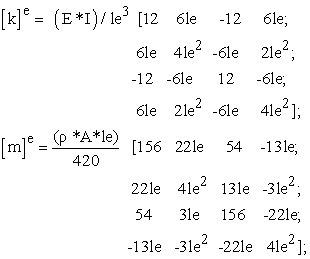where, ‘k’ is element stiffness matrix, ‘E’ is Young’s modulus, ‘I’ is moment of inertia, ‘le’ is element length, ‘A’ is area of cross section, ‘ρ’ represents density of material and ‘e’ represents the element number.

Hereafter, the crack is taken into consideration while assembling the global stiffness matrix.

The element stiffness matrix is later assembled into global stiffness matrix according to the order of elements discretized. In broad-spectrum the value of damping that is considered for general purpose computation was considered in this case as well, damping coefficient was assumed as 0.01 N-S/m. Then the final equation is assembled as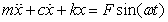.

The equation thus obtained is of higher order and becomes a herculean task to solve a modestly large matrix. Thus, according to the type of support conditions applied to the beam, equivalent boundary conditions are imposed. For diverse supporting condition different type of boundary conditions are applicable.

Consider a beam element of unit length and two nodes at the end.

Here, ‘V’ represents the vertical displacement and ‘θ’ represents rotation, both are combined and represented as nodal displacement vector. Thus, in case of boundary condition either of the term is chosen accordingly to solve the equation. The type of constraints applied are as follows:

•  Free – free: no constraint at any node. The beam can rotate and displace freely.

•  Fixed – free: both displacement and rotation are zero at fixed node. V1=0 and θ1=0.

•  Fixed –fixed: both displacement and rotation at first and last node are zero.V1=0 and θ1=0 and V2=0 and θ2=0

•  Simple supported: Displacement at initial node is zero and rotation at last node is zero. V1=0 and θ2=0.

From the above formulation, the matrix size gets bigger and bigger thereby, integration becomes time consuming when the bandwidth of the matrices becomes large. To improve the computational efficiency, the governing differential equation is transformed into a convenient form by mode superposition method, thereby reducing the bandwidth of the matrix. The differential equations gets decoupled from each other and each of the differential equation is of the second order (linear) and hence it is easy to get their solutions in the closed form.

From the governing differential equation given by,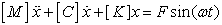(1)

{where [M] is mass matrix, [C] is damping matrix and [K] is global stiffness matrix of size n x n.}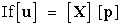(2)

{where [X] is matrix of size n x m of the first m eigen vector (m<<n) and [p] is a generalized displacement vector of size m x 1.}

Then, substituting equation 2 in equation 1 and pre-multiplying with [X]T, we obtain,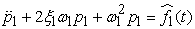(3)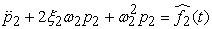(4)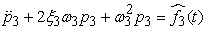(5)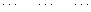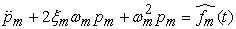(6)

{as [X]T[M][X] is a diagonal matrix of size (m x m) with unity in the principal diagonal, [X]T[C][X] is a diagonal matrix of size m x m whose diagonal elements are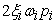(i = 1, 2, 3.., m), [X]T[K][X] is a diagonal matrix with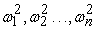(square of natural frequency) in the diagonal and lastly, [X]T[F (t)] of size m x 1 has its elements as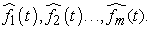When the system is excited, it responds in one or more of its natural modes of vibration, Fundamental or first frequency is represented as the lowest frequency, this gives the start point of the modes. Generally, low frequency waves are the once considered as catastrophic, hence we are generally interested in seeing the first few natural frequencies, and modes associated with it, which carry potential of resonance due to their low frequencies. Besides, other higher order modes are neglected because they are gradually diminishing in the due progress.

By choosing ‘m’ modes only, we are restricting the contribution to first ‘m’ modes assuming that the rest do not contribute to the response. Thus, impact of only first, second and third mode is only considered here.

2.2. HILBERT – HUANG TRANSFORM

Hilbert-Huang Transform (HHT) is an algorithm in which the fundamental part is the empirical mode decomposition (EMD) method. Using the EMD method, any complicated data set can be decomposed into a finite and often small number of components, which is a collection of intrinsic mode functions (IMF).

An IMF represents a generally simple oscillatory mode as a counterpart to the simple harmonic function. By definition, an IMF is any function with the same number of extreme points and zero crossings or at most differ by one in whole data set, and with its envelopes being symmetric with respect to zero or mean value of the envelope defined by local maxima and the envelope defined by the local minima is zero at every point, IMF is complete, adaptive and orthogonal representation.

This decomposition method operating in the time domain is adaptive and highly efficient. Since the decomposition is based on the local characteristic time scale of the data, it can be applied to nonlinear and non-stationary processes.

The EMD algorithm can be summarized as follows:

1. Initialize r0 = x(t) and i = 1;

2. Extract the ith IMF

a. initialize hi(k-1) = ri, k = 1;

b. extract the local maxima and local minima of hi(k-1);

c.interpolate the local maxima and minima by cubic spline to form upper and lower envelopes of hi(k-1);

d.calculate mean mi(k-1) of the upper and lower envelopes of hi(k-1);

e.let hik = hi(k-1) - mi(k-1);

f.if hik is IMF then set IMFi = hik, else go to step ‘b’ with k = k + 1;

3. Define ri+1 = ri – IMFi;

4. If ri+1 still has two extreme points then go to step ‘2’ else decomposition process is completed with ri+1 as residue of the signal.

After obtaining IMF, Hilbert transform is applied to each IMF data, Hilbert transform is defined as the convolution of a signal x(t) with 1/t and can emphasize the local properties of x(t), as follows: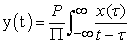{where ‘P’ is the Cauchy principal value}

Coupling x(t) with y(t), we get analytic signal z(t) as: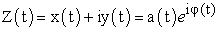{where, a(t) = [x(t) + y(t)]1/2 and φ(t) = arc(tan(y(t)/x(t)))

a(t) is the instantaneous amplitude of x(t) and φ(t) is the instantaneous phase of x(t).

2.3. Assumptions and Limitations of Present Study

Certain assumptions are made in the present analysis while treating joint dynamics. They are:

1) Each layer of the beam undergoes the same transverse deflection.

2) There is no displacement and rotation of the beam at the clamped end.

3) The crack is a non-propagating crack.

### 3. Numerical Results

Results involve calculation of natural frequencies and rotational mode shapes for cantilever beam, simply supported beam, free – free beam and fixed – fixed beam. The first, second and third natural frequencies corresponding to various crack locations are calculated. The fundamental normalized rotational mode shapes for transverse vibration of cracked beams are plotted and compared. All the HHT plots show the results for discretized elements. When the element length (le) is multiplied with the total number of elements (N), we obtain the total length (L) of the beam or alternatively, if the cracked element number is found out, then by multiplying the element length (le) we obtain the distance from either end.

3.1. Theoritical Results
3.1.1. Free - Free Beam (Single Crack)

Input conditions: Aluminum beam E= 6.9e10 Pa, I= 160e-12 m4, L = 0.6m, A= 12e-5m2, rho=2600 Kg/m3,le = 0.015m, N = 40, Crack location is at 18th element, ω1=170.8 rad/sec, ω2= 480.9rad/sec,

This proves the location of crack at 18th element in HHT plot, alternatively, from the element length it can be concluded that the location is 0.27m from left end.

3.1.2. Cantilever Beam (Single Crack)

Input conditions Aluminum beam E= 6.9e10 Pa, I= 312.5e-12 m4, L = 0.4m, A= 15e-5m2, rho=2600 Kg/m3, le = 0.01m, N = 40,ω1=126.6 rad/sec, ω2= 1019.9rad/sec, ω3=2523.4 rad/sec, crack is present at element no 10,

The presence of crack is clearly visible at element 10 in HHT plot. The corresponding location of crack is 0.1m from left side.

3.1.3. Fixed - Fixed Beam (Single Crack)

Input conditions: Aluminum beam E= 6.9e10 Pa, I= 312.5e-12 m4, L = 0.4m, A= 15e-5m2, rho=2600 Kg/m3, le = 0.01m, N = 40, ω1=1025.733 rad/sec, ω2= 2642.52rad/sec, ω3=5355.328 rad/sec, crack is present at 15th element

Hence, the location of crack is proved at 15th elementthe location of crack is 0.15m from left end,

3.1.4. Simply Supported (Single Crack Beam)

Input conditions Aluminum beam E= 6.9e10 Pa, I= 160e-12 m4, L = 0.6m, A= 12e-5m2, rho=2600 Kg/m3, le = 0.01m, N = 40, ω1 = 72.2 rad/sec, ω2 = 444.3 rad/sec, ω3 = 1274.1 rad/sec. the crack is present at 20th element.

Thus, the crack can be located at 20th element or 0.2m from the left end.

3.2. Experimental Results

The pictorial view of experimental setup is shown in pictures. A free- free beam is suspended with the aid of a support. Accelerometers are firmly placed on the beam which in turn are connected to SCADA, this leads to computer that uses LMS test lab software for vibration analysis.

The piezoelectric transducers have a micro integrated circuit present which converts the measured force into voltage. This voltage signal when received by SCADA undergoes three stage process, firstly it is amplified, then later the analog signal is converted into digital signal, and lastly, an on-board computer in the SCADA applies fast Fourier transform to the digital signal and this input is given to a computer connected to the SCADA. The computer coupled to SCADA uses LMS test lab software to view the fast Fourier transform and extract vibration modes from it. The beam is excited with the help of a vibration exciter, an impact hammer. The natural frequencies are measured from the function generator at the point of resonance under the excitation. The specimen is allowed vibrate under 1st and 2nd mode of vibration. The corresponding amplitudes from the experimental results are recorded in computer along the length of the beam. Experimental results for natural frequency, mode shape and frequency response functions (FRF) of transverse vibration at various locations along the length of the beam are recorded.

3.2.1. Free – Free Beam (single Crack)

Input conditions: Aluminum beam E= 6.9e10 Pa, I= 160e-12 m4, L = 0.6m, A= 12e-5m2, rho=2600 Kg/m31=170.177 rad/sec, ω2= 471.258rad/sec, the crack is present at 4th node

Figure 22. HHT plot of first mode of a single-cracked free – free beam for experimental case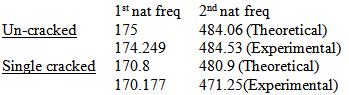First natural frequency of Free- Free beam in theoretical and experimental case matches accurately and second natural frequency is within 5% range of acceptance.

### 4. Concluding Remarks

In this thesis two main contributions were made, firstly, to use a new approach for crack detection and next, to locate crack accurately and swiftly.

It was observed that the natural frequency changes substantially due to the presence of cracks and increase or decrease in the value depends upon the location of crack. The location of the cracks can be predicted from the sudden changes in the spatial variation of the transformed response deviation of the HHT plot.

The experimental analysis shows the effectiveness of the proposed method using HHT towards the identification of location and extent of damage in vibrating structures. And, it is observed that the changes in the vibration signatures become more prominent as the crack grows bigger.

It was learnt while simulating the results that normalized slope mode shapes yield quick and accurate results when compared to the displacement mode shapes and the same has been implemented in this thesis.

### References

  M. Bezhad, A. Ghadami, A. Maghsoodi, J. M. Hale, Vibration based algorithm for crack detection in a cantilever beam containing two different types of crack, Journal of Sound and Vibration 332 (2013) 6312-6320.In article CrossRef  X. B. Lu, J. K. Liu, Z. R. Lu, A two-step approach for crack detection in beam, Journal of Sound and Vibration 332 (2013) 282-293.In article CrossRef  A. P. Adewuyi, Z. Wu, N. H. M. K. Serker, Assessment of vibration based damage identification method using displacement and distributed strain measurement, Structural Health Monitoring (2009) 443-461.In article CrossRef  P. F. Rizo, N. Aspragathos, A. D. Dimarogonas, Identification of crack location and magnitude in a cantilever beam from the vibration modes, Journal of Sound and Vibration 138(3) (1990), 381-388.In article CrossRef  M. Cao, L. Ye, L. Zhou, Z. Su, R. Bai, Sensitivity of fundamental mode shape and static deflection for damage identification in cantilever beams, Mechanical Systems and Signal Processing, 25 (2011) 630-643.In article CrossRef  D. Guo, Z.K. Peng, Vibration analysis of a cracked rotor using Hilbert-Huang transform, Mechanical Systems and Signal Processing, 21 (2007) 3030-3041.In article CrossRef  T. R. Babu, S. Srikanth, A. S. Sekhar, Hilbert-Huang transformation for detection of crack in a transient rotor, Mechanical Systems and Signal Processing, 22 (2008) 905-914.In article CrossRef  B. Li, C. L. Zhang, Hilbert-Huang transformation and its application to crack identification for start-up rotor, Advances in Vibration Engineering 12(5) (2013) 459-473.In article  N. E. Huang, S. S. P. Shen, Hilbert-Huang transform and its applications, World Scientific Publishing Co Pct. Ltd (2005).In article  W. Weaver. Jr., J. M. Gere., Matrix analysis of framed structures, Van Nostrand Reinhold, New York.In article  C. W. de Silva, Vibration fundamentals and practice, CRC Press (2000).In article  M. Feldman, Hilbert transform application in mechanical vibration, John Wiley & Sons, Ltd (2011).In article CrossRef  Z. M. Hussain, A. Z. Sadik, P. O’Shea, Digital signal processing: an introduction with MATLAB and applications, Springer (2011).In article  M. R. Hatch, Vibration simulation using MATLAB and ANSYS, Chapman & Hall/CRC (2001).In article  S. S. Law, Z. R. Lu, Crack identification in beam from dynamic responses, Journal of Sound and Vibration 275 (2005) 967-987.In article CrossRef  Z. K. Peng, Z. Q. Lang, S. A. Billings, Crack detection using nonlinear output frequency response functions, Journal of Sound and Vibration 301 (2007) 777-788.In article CrossRef  S. D. Panteliou, T. G. Chondros, V. C. Argyrakis, Damping factor as an indicator of crack severity, Journal of Sound and Vibration 241(2) (2001) 235-245.In article CrossRef  A. Messina, E. J. Williams, T. Contursi, Structural damage detection by a sensitivity and statistical-based method, Journal of Sound and Vibration 216(5) (1998) 791-808.In article CrossRef